# Prolog Resolution Propositional logic n Propositional logic consists

• Slides: 36Prolog ResolutionPropositional logic n Propositional logic consists of: n The logical values true and false (T and F) n Propositions: “Sentences, ” which n n n Are atomic (that is, they must be treated as indivisible units, with no internal structure), and Have a single logical value, either true or false Operators, both unary and binary; when applied to logical values, yield logical values n The usual operators are and, or, not, and implies 2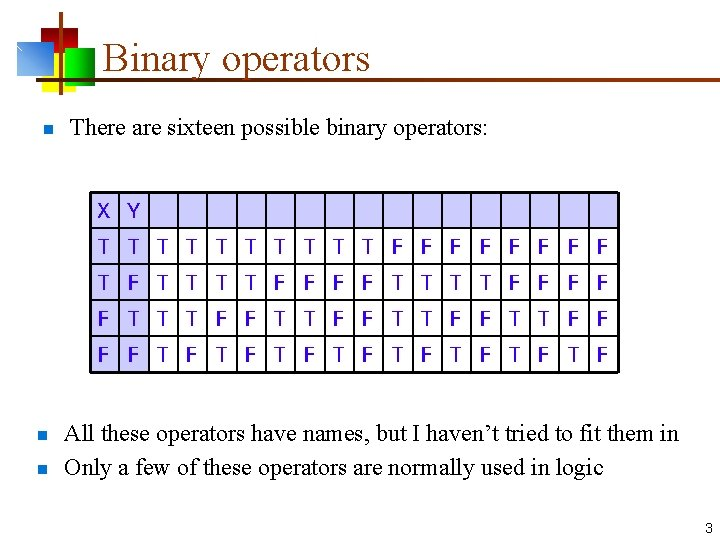Binary operators n There are sixteen possible binary operators: X Y T T T T T F F F F T F T T T T F F F F F T T T F F F F T F T F n n All these operators have names, but I haven’t tried to fit them in Only a few of these operators are normally used in logic 3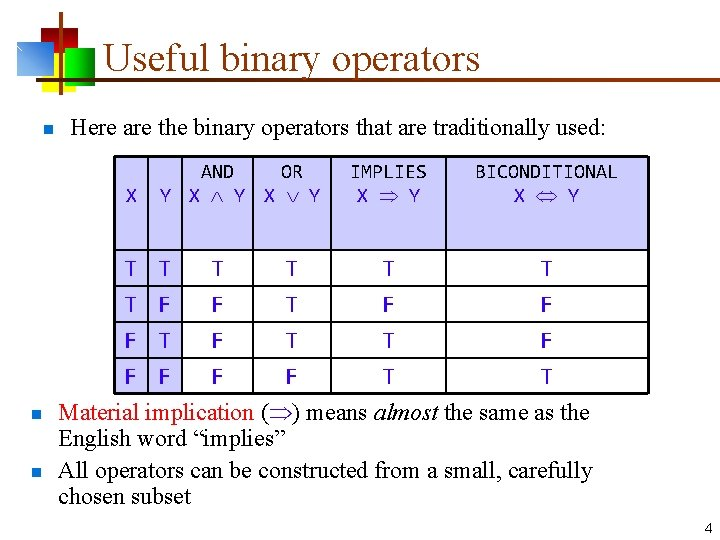Useful binary operators n n n Here are the binary operators that are traditionally used: X AND OR Y X Y T T F F T F T F F F T T T F IMPLIES X Y BICONDITIONAL X Y T F T T T F F T Material implication ( ) means almost the same as the English word “implies” All operators can be constructed from a small, carefully chosen subset 4Logical expressions n n n All logical expressions can be computed with some combination of and ( ), or ( ), and not ( ) operators For example, logical implication can be computed this way: X Y X X Y T T F T T T F F F T T T Notice that X Y is equivalent to X Y 5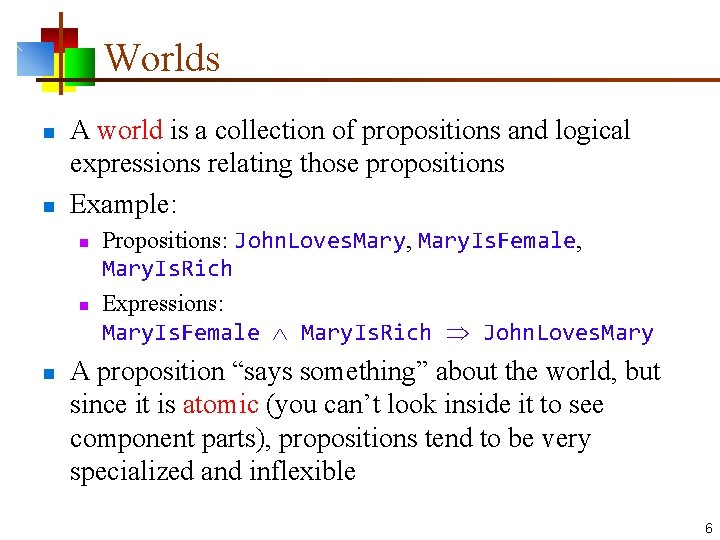Worlds n n A world is a collection of propositions and logical expressions relating those propositions Example: n n n Propositions: John. Loves. Mary, Mary. Is. Female, Mary. Is. Rich Expressions: Mary. Is. Female Mary. Is. Rich John. Loves. Mary A proposition “says something” about the world, but since it is atomic (you can’t look inside it to see component parts), propositions tend to be very specialized and inflexible 6Models A model is an assignment of a truth value to each proposition, for example: n n John. Loves. Mary: T, Mary. Is. Female: T, Mary. Is. Rich: F An expression is satisfiable if there is a model for which the expression is true n For example, the following expression is satisfiable, because the above model makes the expression true: Mary. Is. Female Mary. Is. Rich John. Loves. Mary n An expression is valid if it is satisfied by every model n This expression is not valid: Mary. Is. Female Mary. Is. Rich John. Loves. Mary because it is not satisfied by this model: John. Loves. Mary: F, Mary. Is. Female: T, Mary. Is. Rich: T n But this expression is valid: Mary. Is. Female Mary. Is. Rich Mary. Is. Female 7Inference rules in propositional logic n Here are just a few of the rules you can apply when reasoning in propositional logic: From aima. eecs. berkeley. edu/slides-ppt, chs 7 -9 8Implication elimination n A particularly important rule allows you to get rid of the implication operator, : n n n X Y We will use this later on as a necessary tool for simplifying logical expressions The symbol means “is logically equivalent to” 9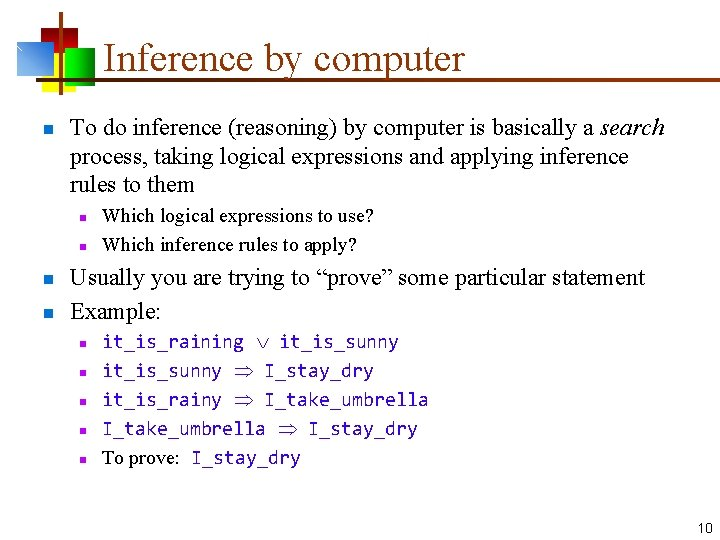Inference by computer n To do inference (reasoning) by computer is basically a search process, taking logical expressions and applying inference rules to them n n Which logical expressions to use? Which inference rules to apply? Usually you are trying to “prove” some particular statement Example: n n n it_is_raining it_is_sunny I_stay_dry it_is_rainy I_take_umbrella I_stay_dry To prove: I_stay_dry 10Conjunction elimination n n Another important rule for simplifying logical expressions allows you to get rid of the conjunction (and) operator, : This rule simply says that if you have an and operator at the top level of a fact (logical expression), you can break the expression up into two separate facts: n Mary. Is. Female Mary. Is. Rich n becomes: n Mary. Is. Female n Mary. Is. Rich 11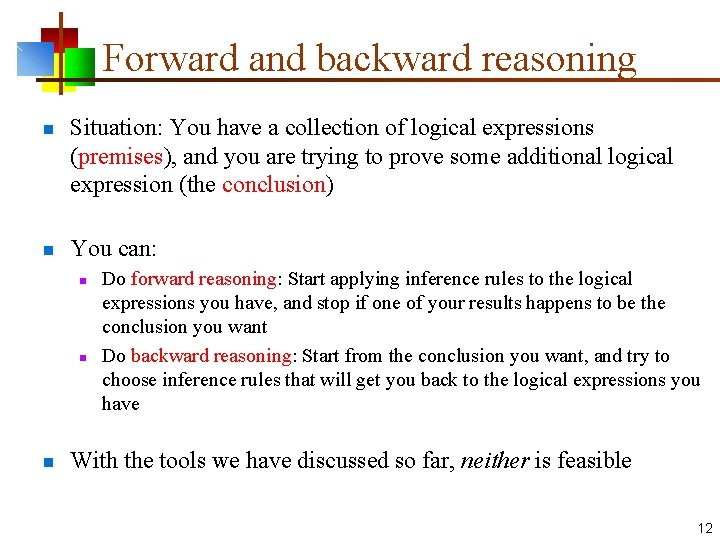Forward and backward reasoning n n Situation: You have a collection of logical expressions (premises), and you are trying to prove some additional logical expression (the conclusion) You can: n n n Do forward reasoning: Start applying inference rules to the logical expressions you have, and stop if one of your results happens to be the conclusion you want Do backward reasoning: Start from the conclusion you want, and try to choose inference rules that will get you back to the logical expressions you have With the tools we have discussed so far, neither is feasible 12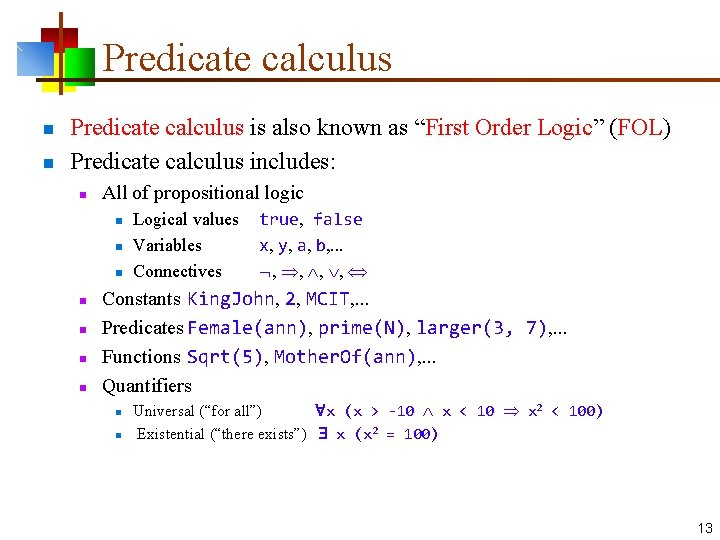Predicate calculus n n Predicate calculus is also known as “First Order Logic” (FOL) Predicate calculus includes: n All of propositional logic n n n n Logical values Variables Connectives true, false x, y, a, b, . . . , , Constants King. John, 2, MCIT, . . . Predicates Female(ann), prime(N), larger(3, 7), . . . Functions Sqrt(5), Mother. Of(ann), . . . Quantifiers n n Universal (“for all”) x (x > -10 x < 10 x 2 < 100) Existential (“there exists”) x (x 2 = 100) 13Constants, functions, and predicates n A constant represents a “thing”--it has no truth value, and it does not occur “bare” in a logical expression n n Given zero or more arguments, a function produces a constant as its value: n n Examples: David. Matuszek, 5, Earth, good. Idea Examples: mother. Of(David. Matuszek), add(2, 2), this. Planet() A predicate is like a function, but produces a truth value n Examples: great. Teacher(David. Matuszek), is. Planet(Earth), greater(3, add(2, 2)) 14Universal quantification n The universal quantifier, , is read as “for each” or “for every” n n Example: x, x 2 0 (for all x, x 2 is greater than or equal to zero) Typically, is the main connective with : x, at(x, University. Of. Pennsylvania) smart(x) means “Everyone at the University of Pennsylvania is smart” n Common mistake: using as the main connective with : x, at(x, University. Of. Pennsylvania) smart(x) means “Everyone is at the University of Pennsylvania and is smart” n If there are no values satisfying the condition, the result is true n Example: x, Person. Is. From. Mars(x) smart(x) is true 15Existential quantification n The existential quantifier, , is read “for some” or “there exists” n n Example: x, x 2 < 0 (there exists an x such that x 2 is less than zero) Typically, is the main connective with : x, at(x, University. Of. Pennsylvania) smart(x) means “There is someone who is at the University of Pennsylvania and who is smart” n Common mistake: using as the main connective with : x, at(x, University. Of. Pennsylvania) smart(x) This is true if there is someone at the University of Pennsylvania who is smart. . . but it is also true if there is someone who is not at the University of Pennsylvania (doesn’t matter whether they are smart or not) By material implication, the result of both F T and F F is T 16Properties of quantifiers n n x y is the same as y x x y is not the same as y x x y Loves(x, y) n n n y x Loves(x, y) n n “There is a person who loves everyone in the world” More exactly: x y (person(x) person(y) Loves(x, y)) “Everyone in the world is loved by at least one person” Quantifier duality: each can be expressed using the other x Likes(x, Ice. Cream) x Likes(x, Broccoli) x Likes(x, Ice. Cream) x Likes(x, Broccoli) From aima. eecs. berkeley. edu/slides-ppt, chs 7 -9 17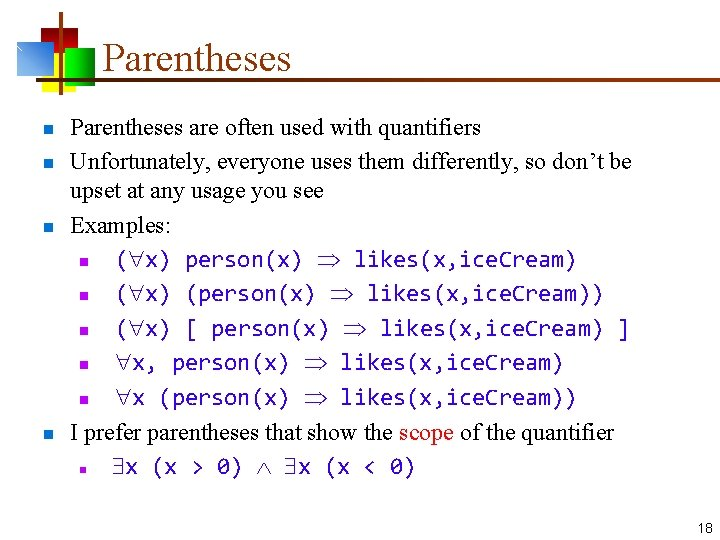Parentheses n n Parentheses are often used with quantifiers Unfortunately, everyone uses them differently, so don’t be upset at any usage you see Examples: n ( x) person(x) likes(x, ice. Cream) n ( x) (person(x) likes(x, ice. Cream)) n ( x) [ person(x) likes(x, ice. Cream) ] n x, person(x) likes(x, ice. Cream) n x (person(x) likes(x, ice. Cream)) I prefer parentheses that show the scope of the quantifier n x (x > 0) x (x < 0) 18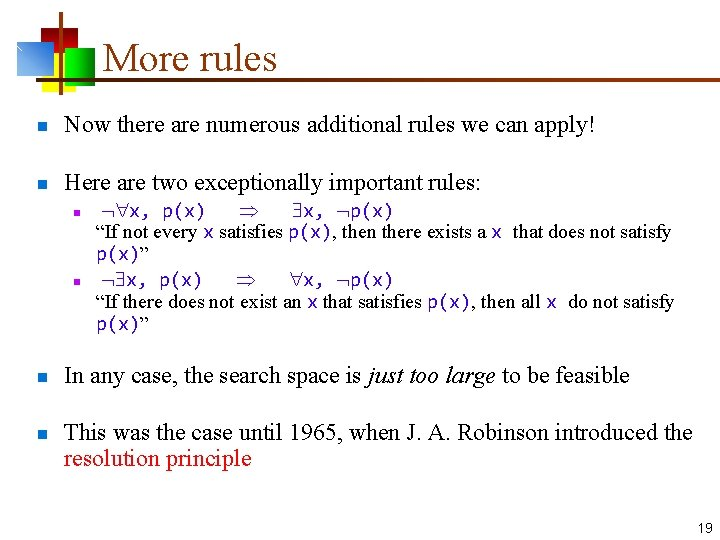More rules n Now there are numerous additional rules we can apply! n Here are two exceptionally important rules: n n x, p(x) x, p(x) “If not every x satisfies p(x), then there exists a x that does not satisfy p(x)” x, p(x) x, p(x) “If there does not exist an x that satisfies p(x), then all x do not satisfy p(x)” In any case, the search space is just too large to be feasible This was the case until 1965, when J. A. Robinson introduced the resolution principle 19Interlude: Definitions n n n syntax: defines the formal structure of sentences semantics: determines the truth of sentences wrt (with respect to) models entailment: one statement entails another if the truth of the first means that the second must also be true inference: deriving sentences from other sentences soundness: derivations produce only entailed sentences completeness: derivations can produce all entailed sentences 20Logic by computer was infeasible n Why is logic so hard? n n You start with a large collection of facts (predicates) You start with a large collection of possible transformations (rules) n n n Some of these rules apply to a single fact to yield a new fact Some of these rules apply to a pair of facts to yield a new fact So at every step you must: n n Choose some rule to apply Choose one or two facts to which you might be able to apply the rule n If there are n facts n n There are n potential ways to apply a single-operand rule There are n * (n - 1) potential ways to apply a two-operand rule Add the new fact to your ever-expanding fact base The search space is huge! 21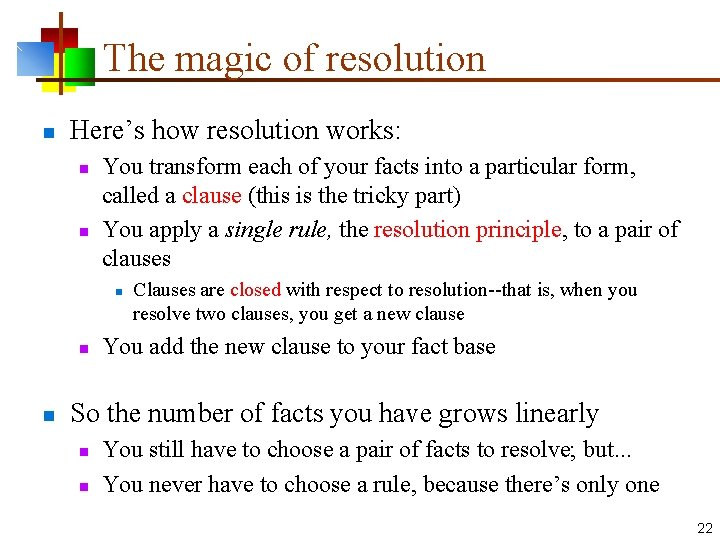The magic of resolution n Here’s how resolution works: n n You transform each of your facts into a particular form, called a clause (this is the tricky part) You apply a single rule, the resolution principle, to a pair of clauses n n n Clauses are closed with respect to resolution--that is, when you resolve two clauses, you get a new clause You add the new clause to your fact base So the number of facts you have grows linearly n n You still have to choose a pair of facts to resolve; but. . . You never have to choose a rule, because there’s only one 22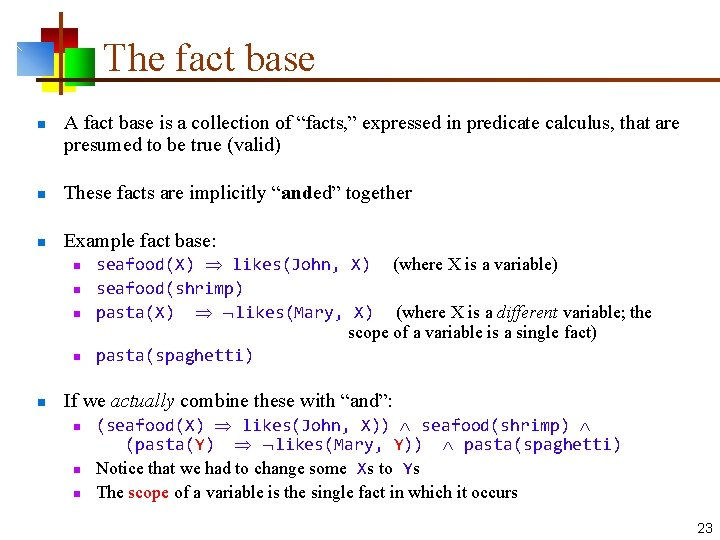The fact base n A fact base is a collection of “facts, ” expressed in predicate calculus, that are presumed to be true (valid) n These facts are implicitly “anded” together n Example fact base: n n n seafood(X) likes(John, X) (where X is a variable) seafood(shrimp) pasta(X) likes(Mary, X) (where X is a different variable; the scope of a variable is a single fact) pasta(spaghetti) If we actually combine these with “and”: n n n (seafood(X) likes(John, X)) seafood(shrimp) (pasta(Y) likes(Mary, Y)) pasta(spaghetti) Notice that we had to change some Xs to Ys The scope of a variable is the single fact in which it occurs 23Clause form n n A clause is a disjunction (“or”) of zero or more literals, some or all of which may be negated Example: sinks(X) dissolves(X, water) ¬denser(X, water) Notice that clauses use only “or” and “not”—they do not use “and, ” “implies, ” or either of the quantifiers “for all” or “there exists” The impressive part is that any predicate calculus expression can be put into clause form n Existential quantifiers, , are the trickiest ones 24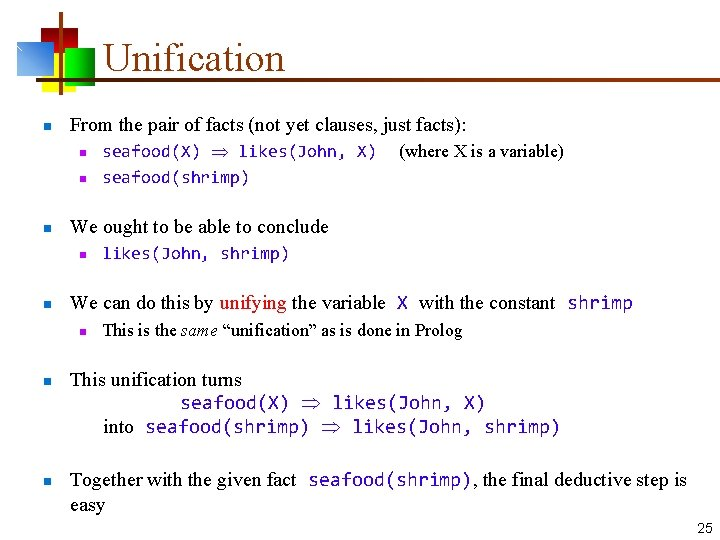Unification n From the pair of facts (not yet clauses, just facts): n n likes(John, shrimp) We can do this by unifying the variable X with the constant shrimp n n (where X is a variable) We ought to be able to conclude n n seafood(X) likes(John, X) seafood(shrimp) This is the same “unification” as is done in Prolog This unification turns seafood(X) likes(John, X) into seafood(shrimp) likes(John, shrimp) Together with the given fact seafood(shrimp), the final deductive step is easy 25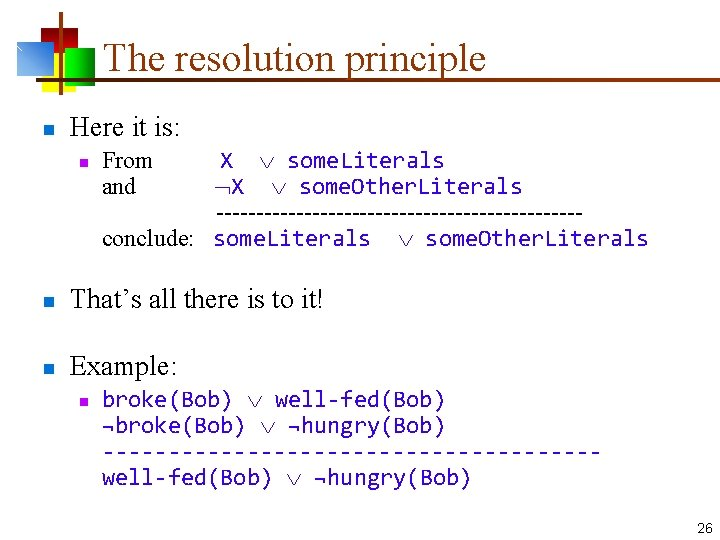The resolution principle n Here it is: n X some. Literals X some. Other. Literals -----------------------conclude: some. Literals some. Other. Literals From and n That’s all there is to it! n Example: n broke(Bob) well-fed(Bob) ¬broke(Bob) ¬hungry(Bob) -------------------well-fed(Bob) ¬hungry(Bob) 26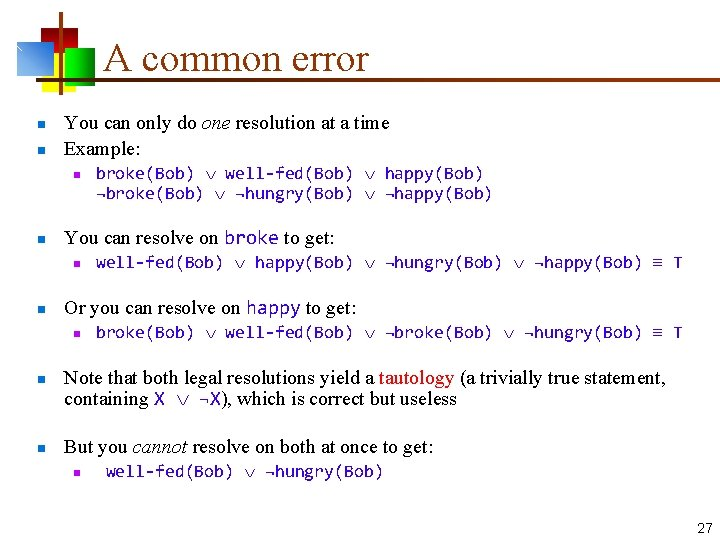A common error n n You can only do one resolution at a time Example: n n You can resolve on broke to get: n n n well-fed(Bob) happy(Bob) ¬hungry(Bob) ¬happy(Bob) T Or you can resolve on happy to get: n n broke(Bob) well-fed(Bob) happy(Bob) ¬broke(Bob) ¬hungry(Bob) ¬happy(Bob) broke(Bob) well-fed(Bob) ¬broke(Bob) ¬hungry(Bob) T Note that both legal resolutions yield a tautology (a trivially true statement, containing X ¬X), which is correct but useless But you cannot resolve on both at once to get: n well-fed(Bob) ¬hungry(Bob) 27Contradiction n n A special case occurs when the result of a resolution (the resolvent) is empty, or “NIL” Example: n n n hungry(Bob) ¬hungry(Bob) --------NIL In this case, the fact base is inconsistent This is a very useful observation when doing resolution theorem proving 28A first example n “Everywhere that John goes, Rover goes. John is at school. ” n n n We use implication elimination to change the first of these into clause form: n n n at(John, X) at(Rover, X) (not yet in clause form) at(John, school) (already in clause form) at(John, X) at(Rover, X) at(John, school) We can resolve these on at(-, -), but to do so we have to unify X with school; this gives: n at(Rover, school) 29Refutation resolution n The previous example was easy because it had very few clauses When we have a lot of clauses, we want to focus our search on the thing we would like to prove We can do this as follows: n n Assume that our fact base is consistent (we can’t derive NIL) Add the negation of the thing we want to prove to the fact base Show that the fact base is now inconsistent Conclude thing we want to prove 30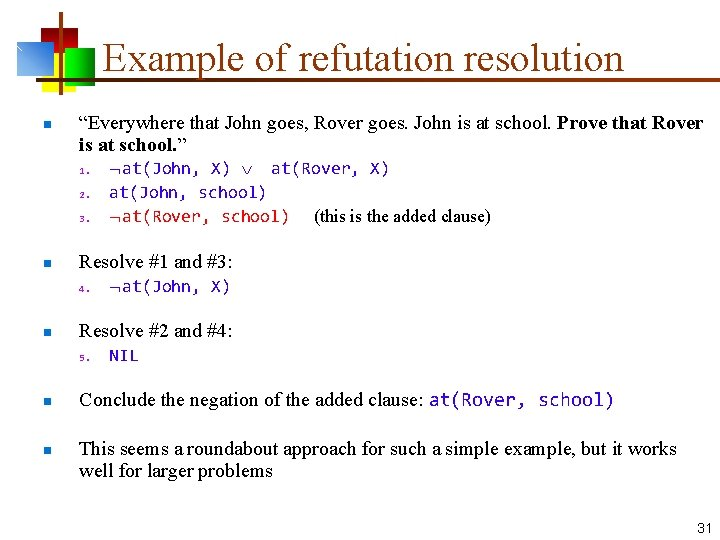Example of refutation resolution n “Everywhere that John goes, Rover goes. John is at school. Prove that Rover is at school. ” 1. 2. 3. n Resolve #1 and #3: 4. n n at(John, X) Resolve #2 and #4: 5. n at(John, X) at(Rover, X) at(John, school) at(Rover, school) (this is the added clause) NIL Conclude the negation of the added clause: at(Rover, school) This seems a roundabout approach for such a simple example, but it works well for larger problems 31Convert to clause form 1. 2. 3. 4. it_is_raining it_is_sunny I_stay_dry it_is_raining I_take_umbrella I_stay_dry 5. 6. 7. 8. it_is_raining it_is_sunny I_stay_dry it_is_raining I_take_umbrella I_stay_dry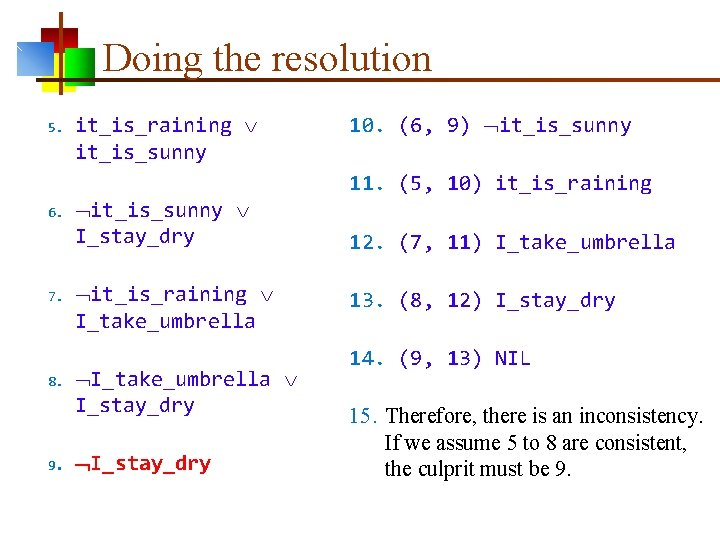Doing the resolution 5. 6. 7. 8. 9. it_is_raining it_is_sunny I_stay_dry it_is_raining I_take_umbrella I_stay_dry 10. (6, 9) it_is_sunny 11. (5, 10) it_is_raining 12. (7, 11) I_take_umbrella 13. (8, 12) I_stay_dry 14. (9, 13) NIL 15. Therefore, there is an inconsistency. If we assume 5 to 8 are consistent, the culprit must be 9.All on one slide n Start with: 1. 2. 3. 4. n 6. 7. 8. n it_is_raining it_is_sunny I_stay_dry it_is_raining I_take_umbrella I_stay_dry Convert to clause form: 5. it_is_raining it_is_sunny I_stay_dry it_is_raining I_take_umbrella I_stay_dry Prove that I stay dry: 9. n I_stay_dry Proof: 10. 11. 12. 13. 14. § (6, 9) it_is_sunny (5, 10) it_is_raining (7, 11) I_take_umbrella (8, 12) it_is_raining (9, 7) NIL Therefore, I_stay_dry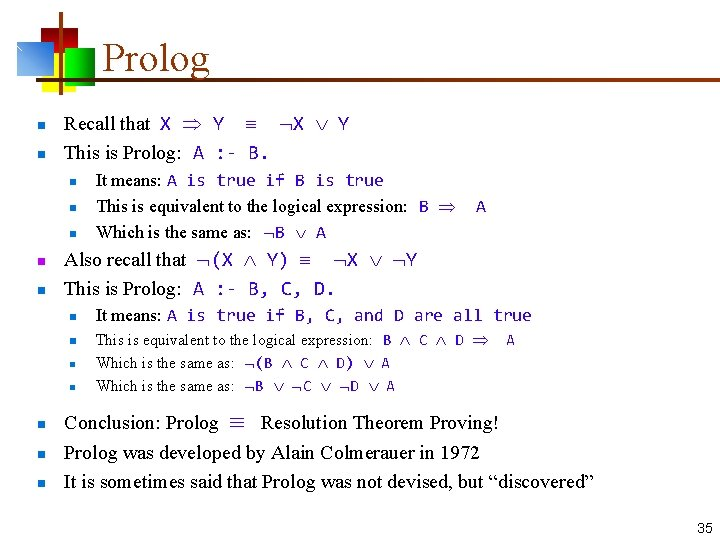Prolog n n Recall that X Y This is Prolog: A : - B. n n n Also recall that (X Y) X Y This is Prolog: A : - B, C, D. n n n n It means: A is true if B is true This is equivalent to the logical expression: B A Which is the same as: B A It means: A is true if B, C, and D are all true This is equivalent to the logical expression: B C D A Which is the same as: (B C D) A Which is the same as: B C D A Conclusion: Prolog Resolution Theorem Proving! Prolog was developed by Alain Colmerauer in 1972 It is sometimes said that Prolog was not devised, but “discovered” 35The End 36